# Grade - math word problems

#### Number of examples found: 5314

• Hugo hasHugo has 12 apples, Julka has seven more than Hugo and six less than Anna. How many apples have Anna and how many Julka?
• Wheel diameter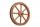A 1m diameter wheel rolled along a 100m long track. How many times did it turn?
• Products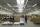15 products are 48 € more expensive than 12 products. How many euros will it cost five same products?
• Angles in triangle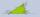Calculate the alpha angle in the triangle if beta is 61 degrees and 98 gamma degrees.
• Balls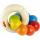The boys changed stamps, beads and balls. For 9 balls is 3 stamps, 2 balls is 44 stamps. How many beads is for 1 ball?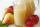Mam used 9 red, 9 green apples and 3 pears to prepare juice. How many pieces of fruit used by mom to prepare this juice?
• Ice cream in coneIn the ice cream cone with a diameter of 5.7 cm is 0.8 dl of ice cream. Calculate the depth of the cone.
• SequenceWrite the first 7 members of an arithmetic sequence: a1=-3, d=6.
• Evaluate expressionCalculate the value of the expression z/3 - 2 z/9 + 1/6, for z = 2
• Ages 2A man's age is 4 times his son's age. After 5 years he will be just twice his son's age, find their ages.
• Expression 6Evaluate expression: -6-2(4-8)-9
• Ounce749 calories in a 7 - ounce serving . What is the unit rate?
• Percent to number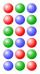To increase that number by 5 percent, we need to multiply it by:
• Can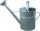Watering can full of water weighs 10 kg. Half-full can weighs 5.5 kg. How much weigh can?
• UN 1If we add to an unknown number his quarter, we get 210. Identify unknown number.
• Wallpaper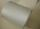Salesman account for 2.3 meters of wallpaper 149.50 Kc. How much cost 1 meter of wallpaper?
• BicycleThe bicycle pedal gear has 36 teeth, the rear gear wheel has 10 teeth. How many times turns rear wheel, when pedal wheel turns 120x?
• EquationHow many real roots has equation ? ?
• Six te 2If 3t-7=5t, then 6t=
• Classroom 3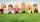How many children are in the classroom, where 13 children are higher than Lenka and 9 children lower than Lenka?

Do you have an interesting mathematical word problem that you can't solve it? Submit a math problem, and we can try to solve it.

We will send a solution to your e-mail address. Solved examples are also published here. Please enter the e-mail correctly and check whether you don't have a full mailbox.

Please do not submit problems from current active competitions such as Mathematical Olympiad, correspondence seminars etc...